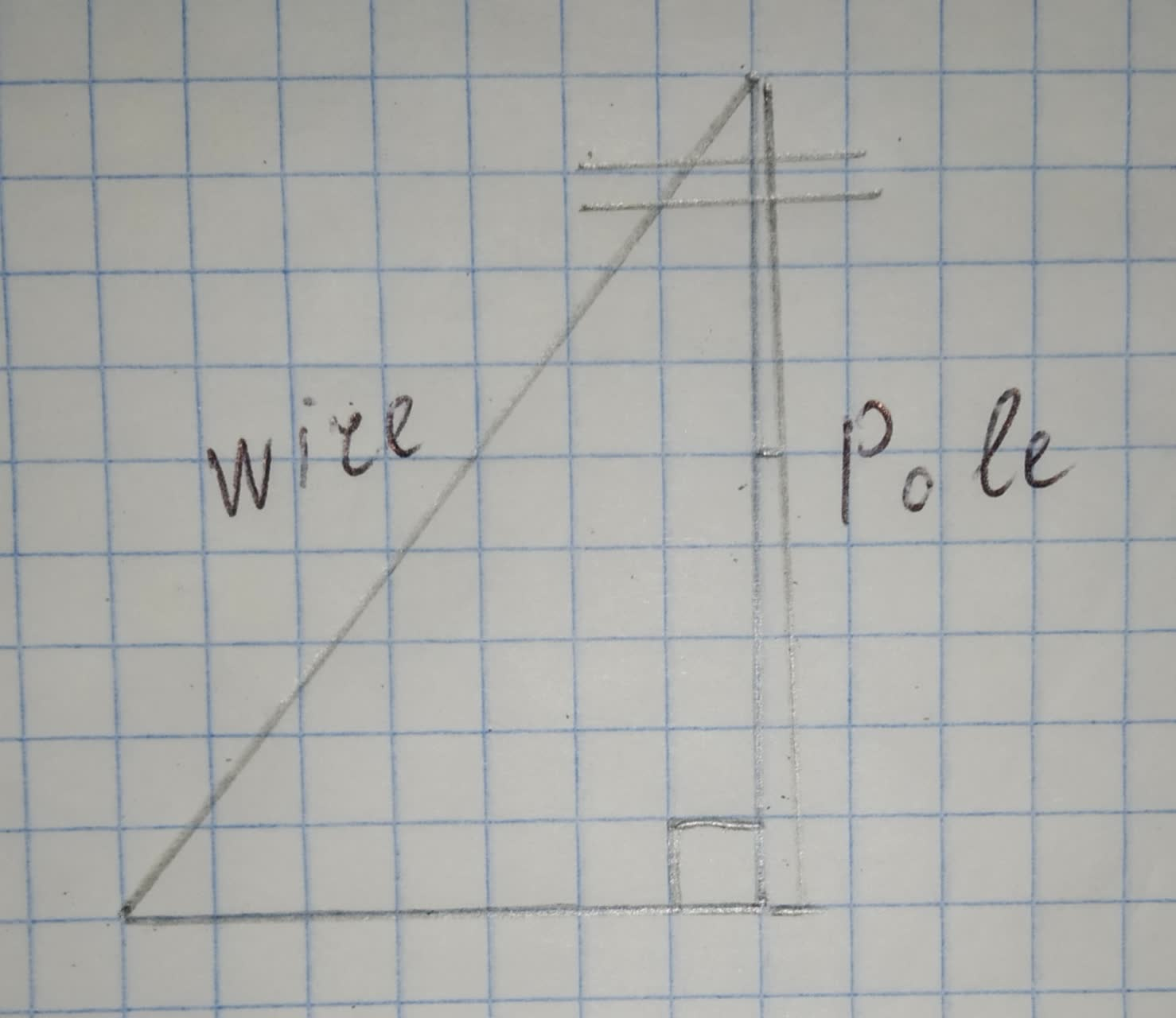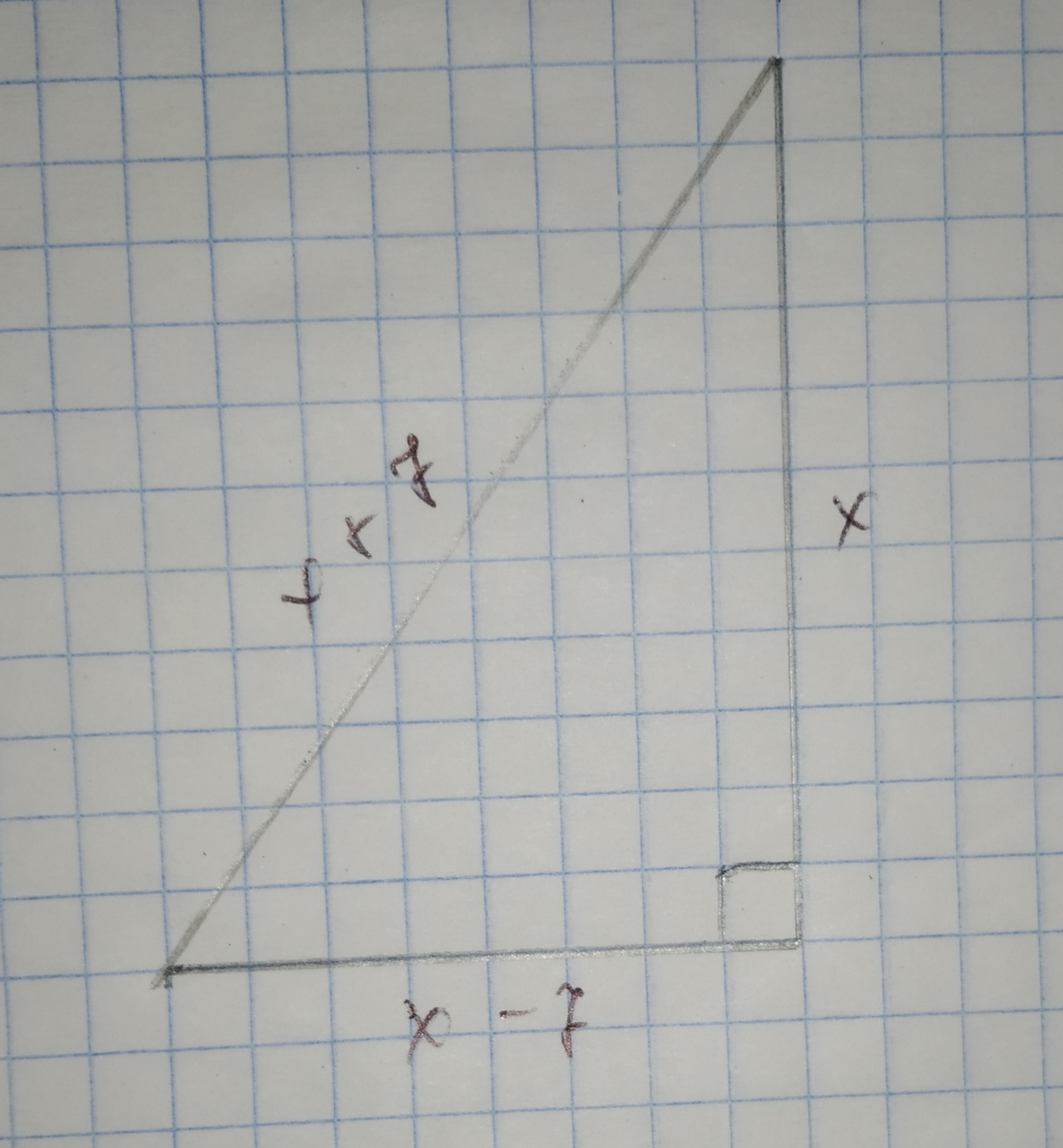# A wire is attached to the top of a pole. The wire is 7 ft longer than the pole, and the distance from the wire to the bottom of the pole is 7 ft lessopatovaL 2020-12-25 Answered
A wire is attached to the top of a pole. The wire is 7 ft longer than the pole, and the distance from the wire to the bottom of the pole is 7 ft less than the height of the pole. Find the length of the wire and the height of the pole.The length of the wire is ? ft, and the height of the pole is ? ft.
You can still ask an expert for help

• Questions are typically answered in as fast as 30 minutes

Solve your problem for the price of one coffee

• Math expert for every subject
• Pay only if we can solve itizboknil3

Step 1
Let x be the height of the pole so that x+7 is the lenght of the wire and the distance from the wire to the bottom of the pole is x - 7, all in ft.
Label the right triangle as shown:Step 2
Using Pythagorean Theorem, we solve for x:
${x}^{2}+{\left(x-7\right)}^{2}={\left(x+7\right)}^{2}$
${x}^{2}+{x}^{2}-14x+49={x}^{2}+14x+49$
$2{x}^{2}-14x+49={x}^{2}+14x+49$
${x}^{2}-28x=0$
Factor:
x(x-28)=0
By zero product property,
x=0,28
The height of the pole cannot be 0 so we tale x = 28 as the solution.
So, the lenght of the wire is 28 + 7 = 35 ft and the height of the pole is 28 ft.
Result:
The lenght of the wire is 35 ft, and the height of the pole is 28 ft.

###### Not exactly what you’re looking for?Jeffrey Jordon